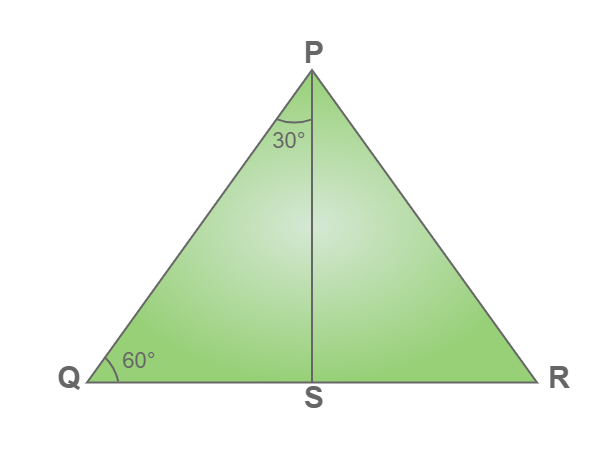Open in App
Not now

# Sin 30 Degrees

• Difficulty Level : Medium
• Last Updated : 17 Sep, 2022

The value of sin 30° is 1/2. In terms of radian sin 30° is written as sin π/6. Trigonometric functions are very important, for various studies such as it is useful to study Wave motion, Movement of light, the study velocity of harmonic oscillators, and other applications. Most common trigonometric functions are the sine function, cosine function, and tangent function.

sin 30° = 1/2 = 0.5

## What is the Value of Sin 30 degrees?

The value of sin 30 degrees is found by various methods, including the formula of the trigonometric ratio as we know that sin x = Perpendicular/Hypotenuse. In a right-angled triangle, ABC with angle A be 30°

Sin 30° = 1/2

## Derivation of Sin 30 Degree (Geometrical Method)

Consider an equilateral triangle PQR where PQ = QR = RP and ∠P = ∠Q = ∠R = 60°. …………….(1)Draw a perpendicular bisector PS of the line QR from vertex P.

Now, QS = RS, and QS = ½ QR = ½ PQ from (1)………(2)

In right angle triangle PQS

sin 30° = Perpendicular / Hypotenuse

here, Perpendicular = QS
Hypotenuse = PQ

sin 30° = QS / PQ

sin 30° = ½ PQ  / PQ

sin 30° = ½

Thus, the value of sin 30° is ½

## Why is the Value of Sin 30 Degree equal to Sin 150 Degree?

Value of sin in the first and second quadrants is positive, whereas it is negative in the third and fourth quadrants.

Value of sin 30° and sin 150° are equal.

sin 30 = sin 150 = ½

We know that sin (180 – θ) = sin θ

hence, sin 150° = sin (180 – 30) = sin 30°

similarly, sin 0 = sin 180 = 0

## Value of Trigonometric Functions

Various values of trigonometric functions are used to solve complex functions. Basic values of trigonometric functions can be learnt from the tale below:

## Solved Examples on Sin 30 degree

Example 1: In a right-angled triangle, the side opposite the angle of 30° is 7m. Find the length of the Hypotenuse.

Solution:

Given: Perpendicular = 7m

Sin 30 = 1/2

P/H = 1/2

7/H = 1/2

H = 7 × 2

H=14m

Example 2: In a right triangle hypotenuse is 20cm, and one side is 10√3cm, find the angles of the triangle.

Solution:

Given: H=20, and B = 10√3

Finding third side using pythagoras theorem.

P2 + B2 = H2

P2 + (10√3)2 = 202

p2 + 300 = 400

P2 = 100

P = 10

The third side is 10cm. The ratio of the third side and the hypotenuse is 1/2 (10/20) So there must be an angle of 30° in triangle Since the triangle is a right angle, so the third angle is

90° – 30° = 60°

The Angles of a triangle are 30°, 60°, 90°.

Example 3: In a right-angled triangle adjacent side is 12 cm and the measure of an angle is 60°. Now find the value of the hypotenuse of the triangle.

Solution:

Given adjacent side = 12 cm

tan 60 = Opposite side/Adjacent side = opposite side/12         (tan 60 = √3)

Now,

1 = Opposite side/12

Opposite side = 12√3 cm

Now,

sin A = Opposite side/Hypotenuse

sin 60 = 10/Hypotenuse

Hypotenuse = 10/sin 60

Hypotenuse = 10/(√3/2)

Hypotenuse = 20√3/3

## FAQs on sin 30 degree

Question 1: Write the value of sine 30°.

The value of sin 30 degrees is 1/2 or 0.5.

Question 2: Write the value of 30 degrees in radian.

The value of 30 degrees in radian is π/6.

Question 3: Write the formula for sin function

In a right-angle triangle sin of an angle, is equal to the ratio of the opposite side of the angle and hypotenuse.

i.e  sin A = Opposite Side/Hypotenuse

Question 4: Write the value of cos 30° and tan 30°.